•  在之前的文中，我们已经进行过一些基本的图像处理。比如，使用低通滤波可以将图像模糊，也有些许降噪的作用。这些都是在空间域内进行的滤波处理，这个处理主要是依靠卷积来进行计算的。首先，从连续的一维卷积入手...
转自http://blog.csdn.net/zhoufan900428/article/details/17194289

1.傅里叶变换与频域

在之前的文中，我们已经进行过一些基本的图像处理。比如，使用低通滤波可以将图像模糊，也有些许降噪的作用。这些都是在空间域内进行的滤波处理，这个处理主要是依靠卷积来进行计算的。首先，从连续的一维卷积入手，如下所示。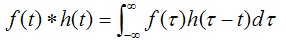将上式进行傅里叶变换，可以得到如下结果。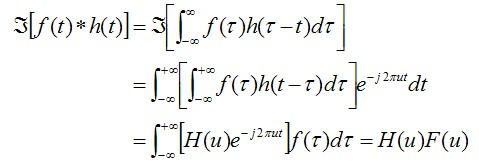从这个式子，我们可以得到一个重要的结论。也就是，函数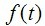与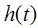卷积的傅里叶变换所得到的结果，是函数与的傅里叶变换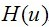与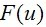的乘积。再将其总结得简单易懂一些，有如下结论。在将其扩展到二维的形况下，假设尺寸为MxN的图像，如下关系是成立的。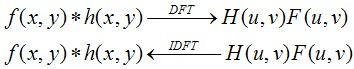其实到这，基本的原理就明了的。我们所看到的图像，均为空间域内的表现形式，我们无法辨识出频域内的图像。要进行频域内的滤波器处理，首先就需要进行傅里叶变换，然后直接进行滤波处理，最后再用反傅里叶变换倒回到空间域内。

到此，已经可以开始空间域内的滤波处理了。但是，还有一点需要注意的地方。使用某个一维信号来举例子，一维信号的傅里叶变换是以2π为周期的函数。所以，我们常常使用的范围[-π,π]来表示这个信号的傅里叶变换，如下所示。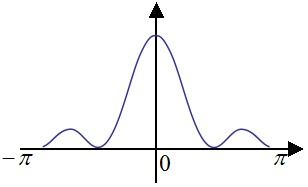这样做的好处是，靠近0的成分就是低频，靠近-π与π的成分就表示高频。而对于图像而言，在Matlab中，我们使用fft2()这个函数来求取图像的傅里叶变换。

[plain] view
plain copyg = fft2(f);

上面这个代码求取的，其实是范围[0,π]内的傅里叶变换。为了方便理解，下图画出了本行代码所求取的图像的傅里叶变换的范围（右）和与其等效的一维傅里叶变换的范围（左）。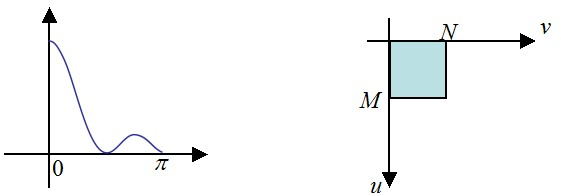很显然，这并不是希望的范围，下面这个代码可以求取[0,2π]内的傅里叶变换。

[plain] view
plain copyP = 2*M;
Q = 2*N;
F = fft2(f,P,Q);

下图画出了本行代码所求取的图像的傅里叶变换的范围（右）和与其等效的一维傅里叶变换的范围（左）。所得到的图像F(u,v)的尺寸为PxQ。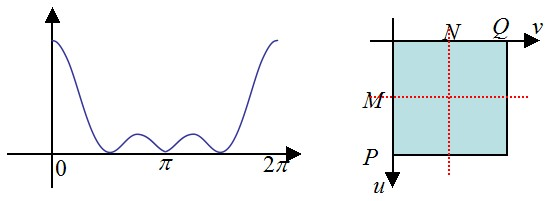我们需要对其移动一下，如下图所示，我们需要的是粉色范围的区域。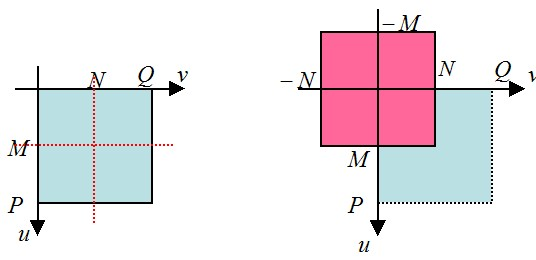下面，从数学上分析一下，如何获得这个部分的频谱。对于傅里叶变换，有如下性质。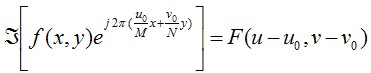这个特性称为平移特性，粉色部分的频谱，将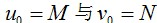带入上式，我们可以得到如下式子。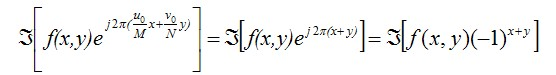为次，我们已经得到了粉色范围的频谱。越靠近傅里叶频谱图像中间的成分，代表了低频成分。其Matlab代码如下所示。

[plain] view
plain copy[M,N] = size(f);
P = 2*M;
Q = 2*N;
fc = zeros(M,N);

for x = 1:1:M
for y = 1:1:N
fc(x,y) = f(x,y) * (-1)^(x+y);
end
end

F = fft2(fc,P,Q);

代码所得到的结果，如下图所示。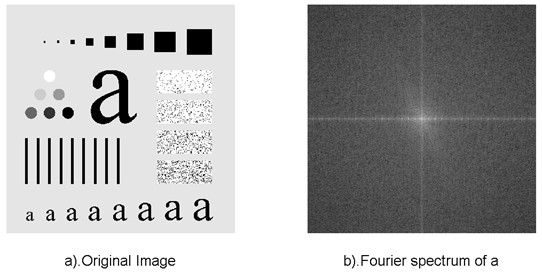接下来，我们总结一下频域滤波的步骤：

①：先将图像做频域内的水平移动，然后求原图像f(x,y)的DFT，得到其图像的傅里叶谱F(u,v)。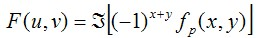②：与频域滤波器做乘积，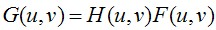③：求取G(u,v)的IDFT，然后再将图像做频域内的水平移动（移动回去），其结果可能存在寄生的虚数，此时忽略即可。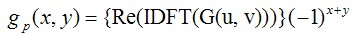④：这里使用ifft2函数进行IDFT变换，得到的图像的尺寸为PxQ。切取左上角的MxN的图像，就能得到结果了。

2.低通滤波器
2.1理想的低通滤波器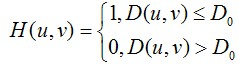其中，D0表示通带的半径。D(u,v)的计算方式也就是两点间的距离，很简单就能得到。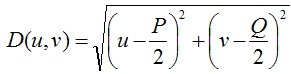使用低通滤波器所得到的结果如下所示。低通滤波器滤除了高频成分，所以使得图像模糊。由于理想低通滤波器的过度特性过于急峻，所以会产生了振铃现象。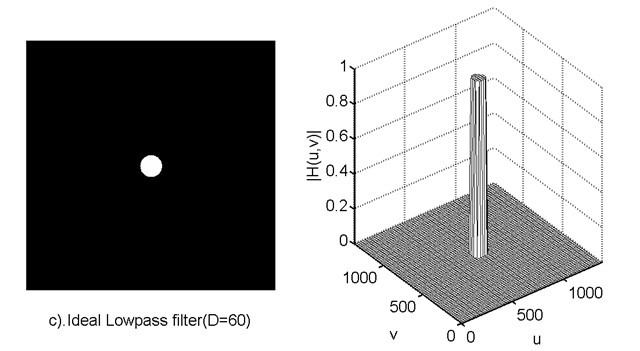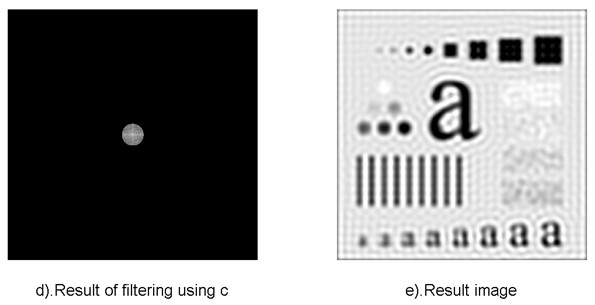2.2巴特沃斯低通滤波器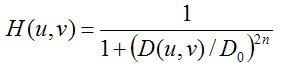同样的，D0表示通带的半径，n表示的是巴特沃斯滤波器的次数。随着次数的增加，振铃现象会越来越明显。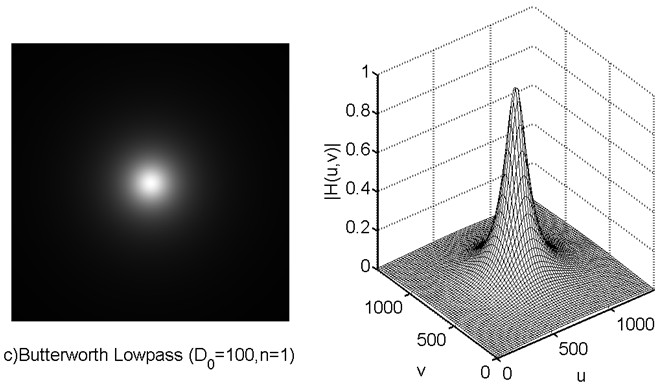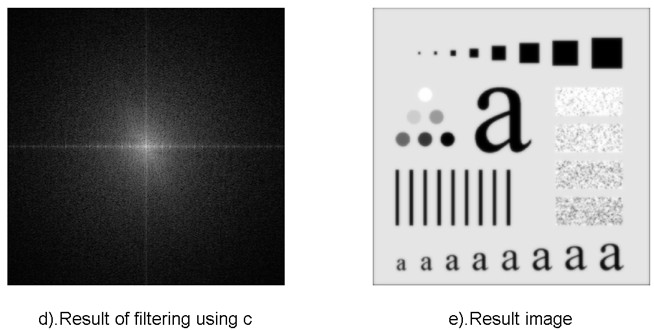2.3高斯低通滤波器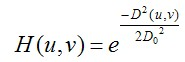D0表示通带的半径。高斯滤波器的过度特性非常平坦，因此是不会产生振铃现象的。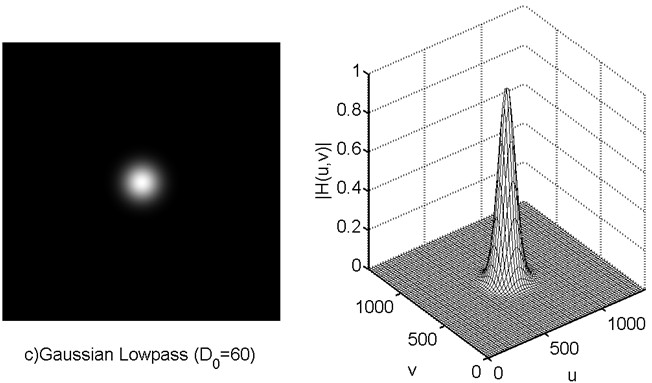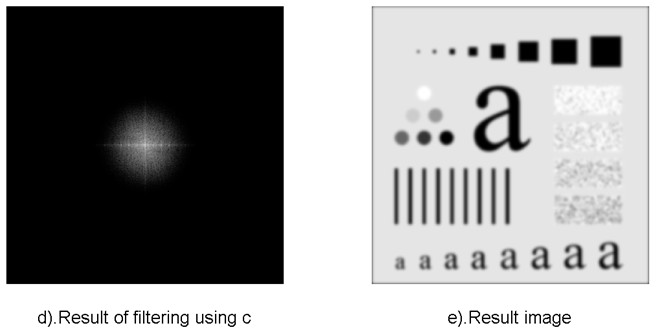3.实现代码

1
2
3
4
5
6
7
8
9
10
11
12
13
14
15
16
17
18
19
20
21
22
23
24
25
26
27
28
29
30
31
32
33
34
35
36
37
38
39
40
41
42
43
44
45
46
47
48
49
50
51
52
53
54
55
56
57
58
59
60
61
62
63
64
65
66
67
68
69
70
71
72
73
74
75
76
77
78
79
80
81
82
83
84
85
86
87
88
89
90
91
92
93
94
95
96
97
98
99
100

close all;clear all;
%% ---------Butterworth Lowpass Filters (Fre. Domain)------------f = imread('characters_test_pattern.tif');f = mat2gray(f,[0 255]);
[M,N] = size(f);P = 2*M;Q = 2*N;fc = zeros(M,N);
for x = 1:1:M    for y = 1:1:N        fc(x,y) = f(x,y) * (-1)^(x+y);    endend
F = fft2(fc,P,Q);
H_1 = zeros(P,Q);H_2 = zeros(P,Q);
for x = (-P/2):1:(P/2)-1     for y = (-Q/2):1:(Q/2)-1        D = (x^2 + y^2)^(0.5);        D_0 = 100;        H_1(x+(P/2)+1,y+(Q/2)+1) = 1/(1+(D/D_0)^2);           H_2(x+(P/2)+1,y+(Q/2)+1) = 1/(1+(D/D_0)^6);     endend

G_1 = H_1 .* F;G_2 = H_2 .* F;
g_1 = real(ifft2(G_1));g_1 = g_1(1:1:M,1:1:N);
g_2 = real(ifft2(G_2));g_2 = g_2(1:1:M,1:1:N);
for x = 1:1:M    for y = 1:1:N        g_1(x,y) = g_1(x,y) * (-1)^(x+y);        g_2(x,y) = g_2(x,y) * (-1)^(x+y);    endend
%% -----show-------figure();subplot(1,2,1);imshow(f,[0 1]);xlabel('a).Original Image');
subplot(1,2,2);imshow(log(1 + abs(F)),[ ]);xlabel('b).Fourier spectrum of a');
figure();subplot(1,2,1);imshow(H_1,[0 1]);xlabel('c)Butterworth Lowpass (D_{0}=100,n=1)');
subplot(1,2,2);h = mesh(1:20:P,1:20:Q,H_1(1:20:P,1:20:Q));set(h,'EdgeColor','k');axis([0 P 0 Q 0 1]);xlabel('u');ylabel('v');zlabel('|H(u,v)|');
figure();subplot(1,2,1);imshow(log(1 + abs(G_1)),[ ]);xlabel('d).Result of filtering using c');
subplot(1,2,2);imshow(g_1,[0 1]);xlabel('e).Result image');
figure();subplot(1,2,1);imshow(H_2,[0 1]);xlabel('f).Butterworth Lowpass (D_{0}=100,n=3)');
subplot(1,2,2);h = mesh(1:20:P,1:20:Q,H_2(1:20:P,1:20:Q));set(h,'EdgeColor','k');axis([0 P 0 Q 0 1]);xlabel('u');ylabel('v');zlabel('|H(u,v)|');
figure();subplot(1,2,1);imshow(log(1 + abs(G_2)),[ ]);xlabel('g).Result of filtering using e');
subplot(1,2,2);imshow(g_2,[0 1]);xlabel('h).Result image');

来自CODE的代码片
Butterworth_Lowpass_Filters.m

1
2
3
4
5
6
7
8
9
10
11
12
13
14
15
16
17
18
19
20
21
22
23
24
25
26
27
28
29
30
31
32
33
34
35
36
37
38
39
40
41
42
43
44
45
46
47
48
49
50
51
52
53
54
55
56
57
58
59
60
61
62
63
64
65
66
67
68
69
70
71
72
73
74
75
76
77
78
79
80
81
82
83
84
85
86
87
88
89
90
91
92
93
94
95
96
97
98
99
100
101
102
103

close all;clear all;clc;%% ---------Gaussian Lowpass Filters (Fre. Domain)------------f = imread('characters_test_pattern.tif');f = mat2gray(f,[0 255]);
[M,N] = size(f);P = 2*M;Q = 2*N;fc = zeros(M,N);
for x = 1:1:M    for y = 1:1:N        fc(x,y) = f(x,y) * (-1)^(x+y);    endend
F = fft2(fc,P,Q);
H_1 = zeros(P,Q);H_2 = zeros(P,Q);
for x = (-P/2):1:(P/2)-1     for y = (-Q/2):1:(Q/2)-1        D = (x^2 + y^2)^(0.5);        D_0 = 60;        H_1(x+(P/2)+1,y+(Q/2)+1) = exp(-(D*D)/(2*D_0*D_0));           D_0 = 160;        H_2(x+(P/2)+1,y+(Q/2)+1) = exp(-(D*D)/(2*D_0*D_0));     endend
G_1 = H_1 .* F;G_2 = H_2 .* F;
g_1 = real(ifft2(G_1));g_1 = g_1(1:1:M,1:1:N);
g_2 = real(ifft2(G_2));g_2 = g_2(1:1:M,1:1:N);
for x = 1:1:M    for y = 1:1:N        g_1(x,y) = g_1(x,y) * (-1)^(x+y);        g_2(x,y) = g_2(x,y) * (-1)^(x+y);    endend

%% -----show-------close all;
figure();subplot(1,2,1);imshow(f,[0 1]);xlabel('a).Original Image');
subplot(1,2,2);imshow(log(1 + abs(F)),[ ]);xlabel('b).Fourier spectrum of a');
figure();subplot(1,2,1);imshow(H_1,[0 1]);xlabel('c)Gaussian Lowpass (D_{0}=60)');
subplot(1,2,2);h = mesh(1:20:P,1:20:Q,H_1(1:20:P,1:20:Q));set(h,'EdgeColor','k');axis([0 P 0 Q 0 1]);xlabel('u');ylabel('v');zlabel('|H(u,v)|');
figure();subplot(1,2,1);imshow(log(1 + abs(G_1)),[ ]);xlabel('d).Result of filtering using c');
subplot(1,2,2);imshow(g_1,[0 1]);xlabel('e).Result image');
figure();subplot(1,2,1);imshow(H_2,[0 1]);xlabel('f).Gaussian Lowpass (D_{0}=160)');
subplot(1,2,2);h = mesh(1:20:P,1:20:Q,H_2(1:20:P,1:20:Q));set(h,'EdgeColor','k');axis([0 P 0 Q 0 1]);xlabel('u');ylabel('v');zlabel('|H(u,v)|');
figure();subplot(1,2,1);imshow(log(1 + abs(G_2)),[ ]);xlabel('g).Result of filtering using e');
subplot(1,2,2);imshow(g_2,[0 1]);xlabel('h).Result image');

来自CODE的代码片
Gaussian_Lowpass_Filters.m

1
2
3
4
5
6
7
8
9
10
11
12
13
14
15
16
17
18
19
20
21
22
23
24
25
26
27
28
29
30
31
32
33
34
35
36
37
38
39
40
41
42
43
44
45
46
47
48
49
50
51
52
53
54
55
56
57
58
59
60
61
62
63
64
65
66
67
68
69
70
71
72
73
74
75
76
77
78
79
80
81
82
83
84
85
86
87
88
89
90
91
92
93
94
95
96
97
98

close all;clear all;
%% ---------Ideal Lowpass Filters (Fre. Domain)------------f = imread('characters_test_pattern.tif');f = mat2gray(f,[0 255]);
[M,N] = size(f);P = 2*M;Q = 2*N;fc = zeros(M,N);
for x = 1:1:M    for y = 1:1:N        fc(x,y) = f(x,y) * (-1)^(x+y);    endend
F = fft2(fc,P,Q);
H_1 = zeros(P,Q);H_2 = zeros(P,Q);
for x = (-P/2):1:(P/2)-1     for y = (-Q/2):1:(Q/2)-1        D = (x^2 + y^2)^(0.5);        if(D <= 60)  H_1(x+(P/2)+1,y+(Q/2)+1) = 1; end            if(D <= 160)  H_2(x+(P/2)+1,y+(Q/2)+1) = 1; end     endend
G_1 = H_1 .* F;G_2 = H_2 .* F;
g_1 = real(ifft2(G_1));g_1 = g_1(1:1:M,1:1:N);
g_2 = real(ifft2(G_2));g_2 = g_2(1:1:M,1:1:N);
for x = 1:1:M    for y = 1:1:N        g_1(x,y) = g_1(x,y) * (-1)^(x+y);        g_2(x,y) = g_2(x,y) * (-1)^(x+y);    endend
%% -----show-------figure();subplot(1,2,1);imshow(f,[0 1]);xlabel('a).Original Image');
subplot(1,2,2);imshow(log(1 + abs(F)),[ ]);xlabel('b).Fourier spectrum of a');
figure();subplot(1,2,1);imshow(H_1,[0 1]);xlabel('c).Ideal Lowpass filter(D=60)');
subplot(1,2,2);h = mesh(1:20:P,1:20:Q,H_1(1:20:P,1:20:Q));set(h,'EdgeColor','k');axis([0 P 0 Q 0 1]);xlabel('u');ylabel('v');zlabel('|H(u,v)|');
figure();subplot(1,2,1);imshow(log(1 + abs(G_1)),[ ]);xlabel('d).Result of filtering using c');
subplot(1,2,2);imshow(g_1,[0 1]);xlabel('e).Result image');
figure();subplot(1,2,1);imshow(H_2,[0 1]);xlabel('f).Ideal Lowpass filter(D=160)');
subplot(1,2,2);h = mesh(1:20:P,1:20:Q,H_2(1:20:P,1:20:Q));set(h,'EdgeColor','k');axis([0 P 0 Q 0 1]);xlabel('u');ylabel('v');zlabel('|H(u,v)|');
figure();subplot(1,2,1);imshow(log(1 + abs(G_2)),[ ]);xlabel('g).Result of filtering using e');
subplot(1,2,2);imshow(g_2,[0 1]);xlabel('h).Result image');


展开全文图像处理 傅里叶变换 低通滤波器
• 最近在看OpenCV 图像处理-平滑处理这个章节，于是呢，想着滤波器总结一下。这里主要是空间域滤波器的介绍，频率域以后再说吧。 首先分为平滑空间滤波器和锐化空间滤波器来进行介绍。平滑空间滤波器主要用于模糊处理...
    最近在看OpenCV 图像处理-平滑处理这个章节，于是呢，想着滤波器总结一下。这里主要是空间域滤波器的介绍，频率域以后再说吧。
首先分为平滑空间滤波器和锐化空间滤波器来进行介绍。平滑空间滤波器主要用于模糊处理和降低噪声，主要两类：均值滤波器和中值滤波器。锐化空间滤波器主要是：拉普拉斯算子和梯度算子
1、均值滤波器
假设我们有一个3*3的模板，系数全为1，那么欲求的中心点像素值就是以该模板为中心的9个像素的平均值来代替。根据定义可知，该滤波器具有模糊边缘的负面效应。通过空间均值处理来模糊图像，可以突出感兴趣的物体，即将较部分图像融入背景中 ，部分图像‘突出显示’出来，达到平滑图像的作用，重点理解平滑对于图像的意义。MATLAB实现代码：

set(gca,'Fontname','Monospaced');
subplot(231),imshow(I), title('origin image');
%I=rgb2gray(I);
%I1=imnoise(I,'salt & pepper',0.02);
%subplot(2 3 2)
%imshow(I1)
%title('添加椒盐噪声的图像');
I1 = I;
k1=filter2(fspecial('average',3),I1)/255; %进行3*3模板平滑滤波
k2=filter2(fspecial('average',5),I1)/255; %进行5*5模板平滑滤波
k3=filter2(fspecial('average',7),I1)/255; %进行7*7模板平滑滤波
k4=filter2(fspecial('average',9),I1)/255; %进行9*9模板平滑滤波
k5=filter2(fspecial('average',15),I1)/255; %进行15*15模板平滑滤波
subplot(232),imshow(k1);title('3*3 smoothing filtering');
subplot(233),imshow(k2);title('5*5 smoothing filtering');
subplot(234),imshow(k3);title('7*7 smoothing filtering');
subplot(235),imshow(k4);title('9*9 smoothing filtering');
subplot(236),imshow(k5);title('15*15 smoothing filtering');  结果：可以看出，在模板逐渐扩大的过程中，图像的锯齿边缘被模糊掉，颗粒状的噪声也没有了，达到了我们想要的效果，但是在9*9甚至更大的模板，图像开始失真，图像过度模糊了。所以我们在图像平滑的时候，要选最佳的模板尺寸。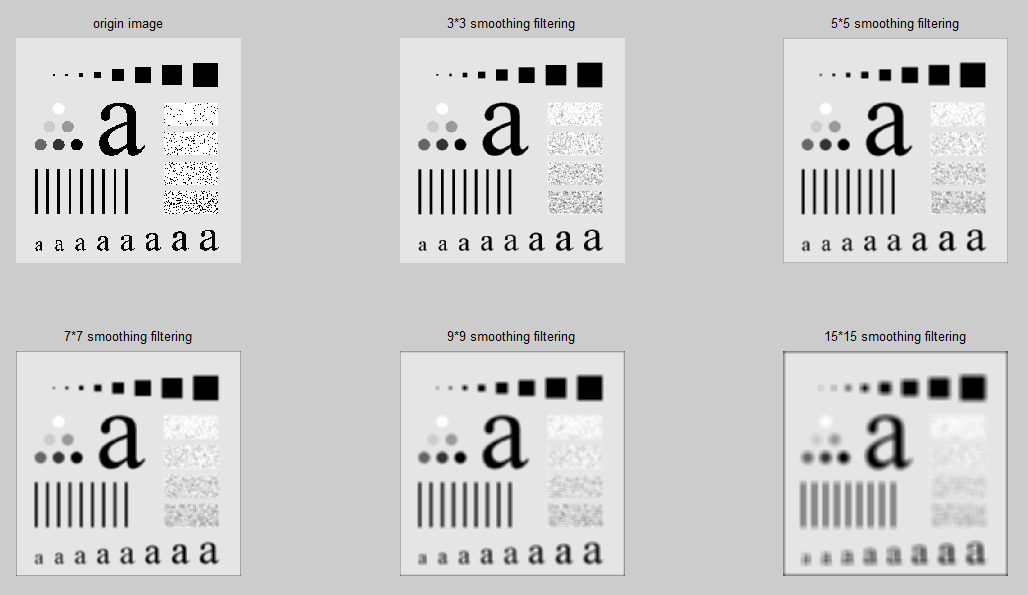2、中值滤波器
中值滤波器是一种统计排序滤波器，即图像像素等于周围像素排序后的中值，加入模板为3*3，则目标点的像素等于排序后的第五个像素的值。中值滤波器特别适用于椒盐噪声。由定义知，中值滤波器对于孤立点特别敏感，还可以保持图像的边缘特性。由中值滤波扩展出来的还有最大值滤波器和最小值滤波器。
MATLAB代码：

%=======second part======
subplot(131),imshow(I);title('原椒盐噪声图像');

k1=filter2(fspecial('average',3),I)/255; 	%进行3*3模板平滑滤波

k2=medfilt2(I,[3,3]); 						%进行3*3模板中值滤波

subplot(132),imshow(k1);title('3*3模板均值滤波');
subplot(133),imshow(k2);title('3*3模板中值滤波');
I(100:110,100:110),
k2(100:110,100:110),结果：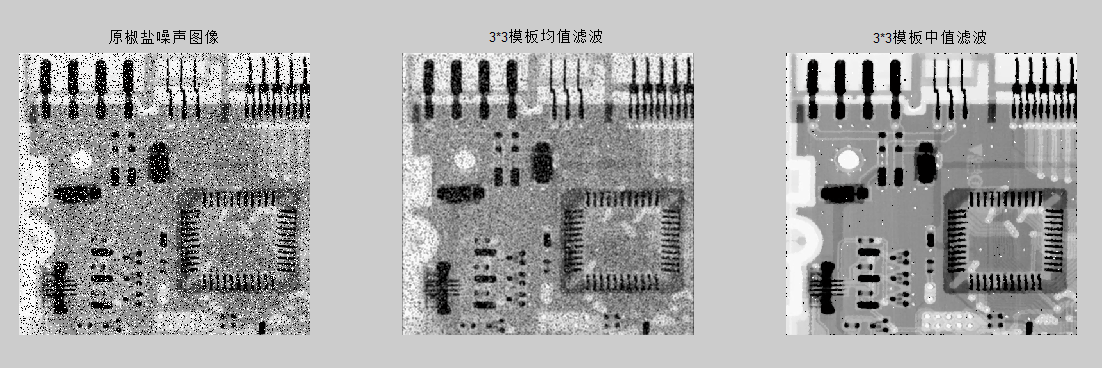增加：
这里补充说下高斯滤波器，高斯滤波器是利用高斯核与输入图像的每个点进行卷积。提到高斯，就想到‘草帽’，更能记住它。

高斯滤波器是一种平滑线性滤波器，使用高斯滤波器对图像进行滤波，其效果是降低图像灰度的“尖锐”变化，也就是使图像“模糊”了。高斯滤波对于抑制服从正态分布的噪声效果非常好，其代价是使图像变得“模糊”。当然，有时对图像进行平滑滤波的目的就是让图像变得模糊。

一维高斯分布函数：                                                                                二维高斯分布函数：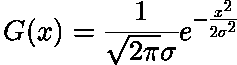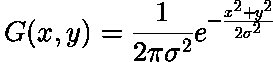它们的图像分别为：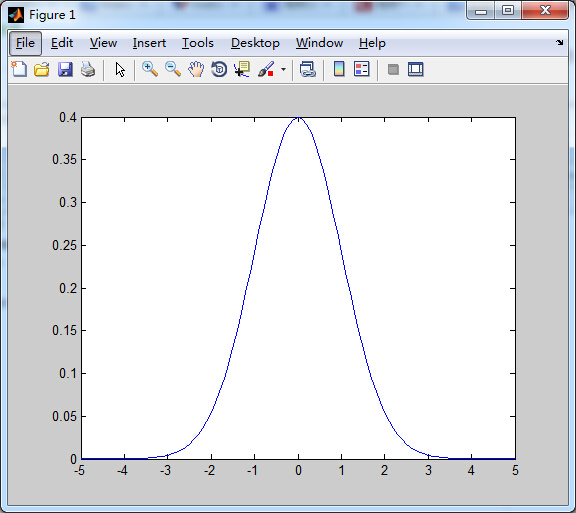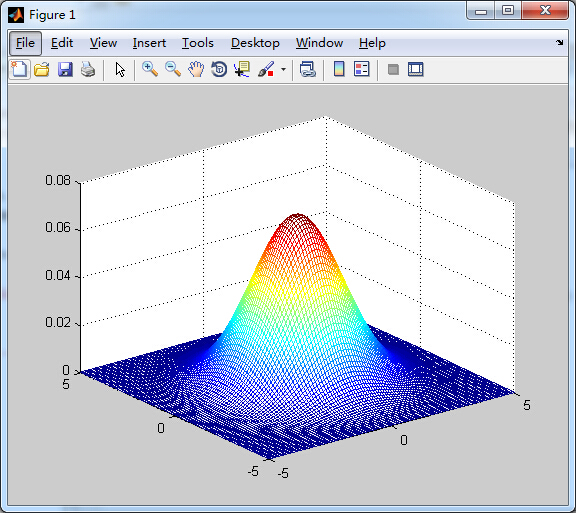其3*3与5*5的卷积核对应如下：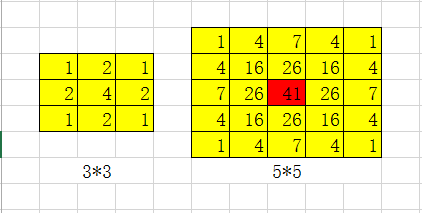MATLAB实现：

img = imread('lena.tif');
w = fspecial('gaussian',[5,5],0.5);
%replicate:图像大小通过赋值外边界的值来扩展
%symmetric 图像大小通过沿自身的边界进行镜像映射扩展
I = imfilter(img,w,'replicate');
subplot(1,2,1);title('原图像');imshow(img);
subplot(1,2,2);title('高斯滤波后的图像');imshow(I);从结果可以看出，图像整体模糊平滑了，特别是头发和帽子部分，是图像的尖锐变化被降低了吧。

3、拉普拉斯算子进行图像锐化
拉普拉斯算子是一种二阶微分算子，其强调的是图像中灰度的突变，通过将原图像和拉普拉斯图像加在一起，可以达到锐化图像的作用，最终在保留图像的背景信息的前提下从而突出细节。拉普拉斯锐化模板在边缘检测中非常有用。因为图像的边缘就是那些灰度跳变厉害的的区域。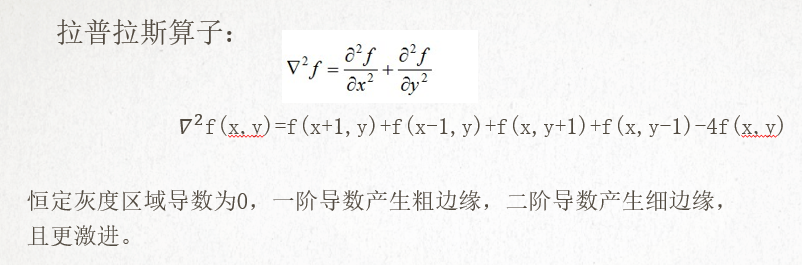拉普拉斯算子模板：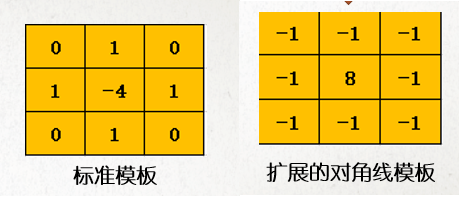实现代码：

%=============================================================
subplot(2,3,1),imshow(f);
title('原图像');

w4=fspecial('laplacian',0),
g1=imfilter(f,w4,'replicate');
subplot(2,3,2),imshow(g1); title('拉普拉斯模板滤波后的图像');

f2=im2double(f);%将f转换归一化的double类图像，然后进行滤波
g2=imfilter(f2,w4,'replicate');
% imshow(img,[low high])  [low high]用于指定图像显示的灰度范围 对于double数据认为是0-1范围内
subplot(2,3,3),imshow(g2,[]);   title('含有负值的滤波结果');

g=f2-g2;
subplot(2,3,4),imshow(g);title('原图-含有负值的滤波结果');

%w8=[0 1 0;1 -4 1;0 1 0],
w8=[1 1 1;1 -8 1;1 1 1],
g8=f - imfilter(f,w8,'replicate');
subplot(2,3,5),imshow(g8);title('对角线中心为8的拉普拉斯模板');

%=============================================================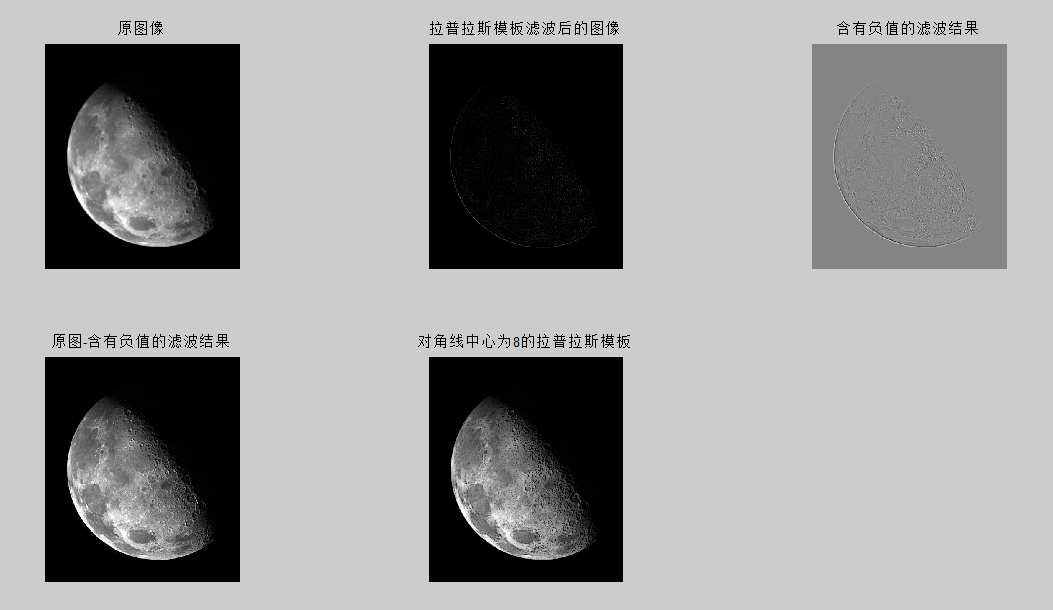4、梯度算子进行图像锐化
相比于拉普拉斯二阶微分算子，梯度算子一一阶微分算子，更为简单。其实个人觉得，把梯度算子放在‘边缘检测’更为合适。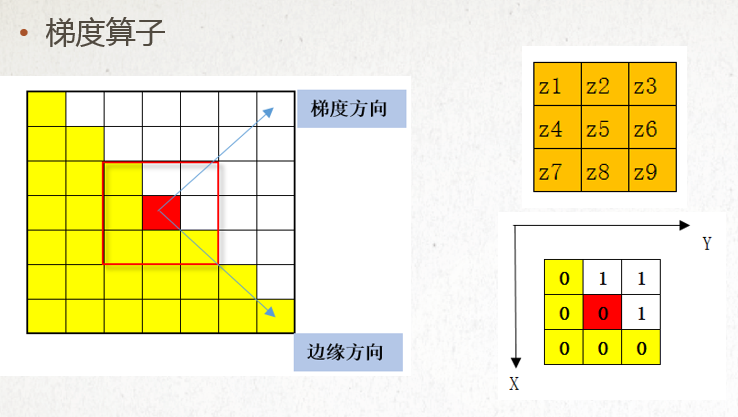f(x,y)在X方向和Y方向的梯度：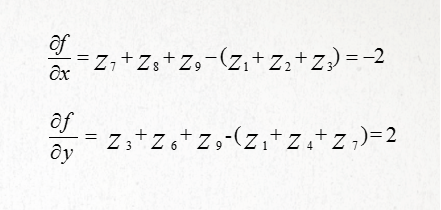经典模板：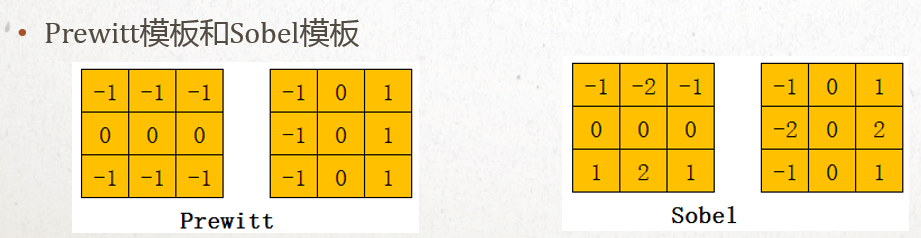代码实现：
代码中增加注释，很详细的介绍了求梯度算子锐化的过程。
%   soble algorithm
%   ZhangFL at SWPU  2017.04.26
%   Sobel算子中的垂直模板得到的梯度图，由于梯度方向与边缘走向垂直，所以该梯度图对水平边缘有较强的响应，从而水平细节信息非常清晰。
%   sobel算子中的水平模板得到的梯度图，它对垂直边缘有较强的响应，垂直细节非常清晰。
%   Sobel算子水平和垂直方向叠加的梯度图，水平和垂直细节都非常清晰。
%I=rgb2gray(A);
I=A;

subplot(2,2,1);
imshow(I);
title('原图');

k2=filter2(fspecial('average',5),I)/255; %进行5*5模板平滑滤波
I=k2;
hx=[-1 -2 -1;0 0 0 ;1 2 1],  %产生sobel垂直梯度模板
hy=hx',                    %产生sobel水平梯度模板

subplot(2,2,2);
title('图像的sobel垂直梯度');

subplot(2,2,3);
title('图像的sobel水平梯度');

subplot(2,2,4);
title('图像的sobel梯度(使用5*5模板平滑滤波)');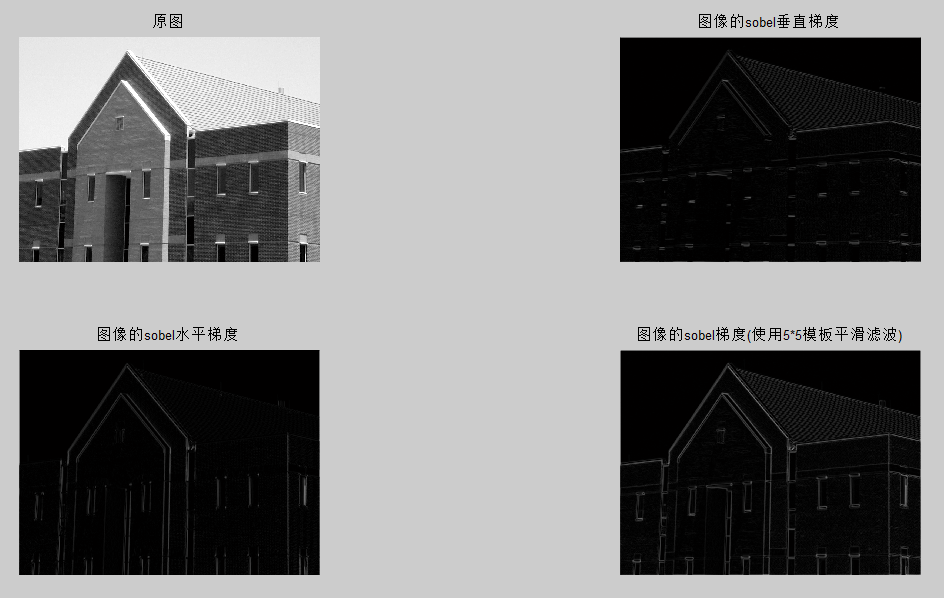展开全文平滑图像 边缘检测
• 本文内容参考《数字图像处理基础》Wilhelm Burger等著。 “点运算”是在不改变图像大小、几何形状以及局部结构的情况下，对像素值进行修改，新图像的像素值只与原图像同一位置的像素值有关。 “滤波器”一般会用到...
本文内容参考《数字图像处理基础》Wilhelm Burger等著。 “点运算”是在不改变图像大小、几何形状以及局部结构的情况下，对像素值进行修改，新图像的像素值只与原图像同一位置的像素值有关。 “滤波器”一般会用到原图像中的多个像素来计算每个新像素，一个滤波器用一个“滤波矩阵”（或“滤波模板”）表示，它的重要参数包括“滤波区域的尺寸”、“滤波区域的形状”。

线性滤波器：线性卷积(可交换性、线性性、结合性、x/y可分离性)

一、平滑滤波器：将图像中某一区域内的像素进行加权平均。
（1.）箱式滤波器： ⎛⎝⎜⎜⎜⎜⎜⎜0000001110011100111000000⎞⎠⎟⎟⎟⎟⎟⎟<!--//--><![CDATA[//><!--
\begin{pmatrix} 0&0&0&0&0\\0&1&1&1&0\\0&1&1&1&0\\0&1&1&1&0\\ 0&0&0&0&0 \end{pmatrix}
//--><!]]>
侧面急剧截断，频域性能不稳定。
低通滤波（削减信号中给定截止频率之上的频率成分）。
（2.）高斯滤波器：
滤波矩阵足够大(5×5<!--//--><![CDATA[//><!--
5\times5
//--><!]]>)相当于离散的二维高斯函数： Gσ(x,y)=e−x2+y22σ2<!--//--><![CDATA[//><!--
G_{\sigma}(x,y)=e^{-\frac{x^2+y^2}{2{\sigma}^2}}
//--><!]]>
“一个二维高斯滤波器”可以分解成“一对一维滤波器”。
（3.）差分滤波器:
滤波矩阵中有一些滤波系数是负数。
差分滤波器的应用：图像的边缘增强、图像锐化。

非线性滤波器：

（1.）最小值与最大值滤波（如，椒盐噪声） I′(u,v)←minI(u+i,v+j)|(i,j)∈R<!--//--><![CDATA[//><!--
I^{'}(u,v)\gets \min{I(u+i,v+j)|(i,j)\in R}
//--><!]]>I′(u,v)←maxI(u+i,v+j)|(i,j)∈R<!--//--><![CDATA[//><!--
I^{'}(u,v)\gets \max{I(u+i,v+j)|(i,j)\in R}
//--><!]]>
其中R<!--//--><![CDATA[//><!--
R
//--><!]]>表示滤波区域，即要用进行滤波的矩阵块坐标集合。
（2.）中值滤波
对应滤波区域R<!--//--><![CDATA[//><!--
R
//--><!]]>内像素值的中值作为对应点的新像素，即将滤波区域的像素值从小到大排序，取排序后的中位数。 median(p0,p1,⋯,pK−1,pK,pK+1,⋯,p2K)=pK<!--//--><![CDATA[//><!--
median(p_{0},p_{1},\cdots,p_{K-1},p_{K},p_{K+1},\cdots,p_{2K})=p_{K}
//--><!]]>
（3.）加权中值滤波
为滤波区域中的每个位置分配一个独立的权值，对应像素值的“投票次数”，记权值矩阵为W(i,j)<!--//--><![CDATA[//><!--
W(i,j)
//--><!]]>。
比如I(u,v)=⎛⎝⎜319705208⎞⎠⎟,W(i,j)=⎛⎝⎜121232121⎞⎠⎟<!--//--><![CDATA[//><!--
I(u,v)=\begin{pmatrix} 3&7&2\\1&0&0\\9&5&8 \end{pmatrix},W(i,j)=\begin{pmatrix} 1&2&1\\2&3&2\\1&2&1 \end{pmatrix}
//--><!]]>
则需要对3,7,7,2,1,1,0,0,0,0,0,9,5,5,8<!--//--><![CDATA[//><!--
3,7,7,2,1,1,0,0,0,0,0,9,5,5,8
//--><!]]>进行排序取中位数，得pk=2<!--//--><![CDATA[//><!--
p_{k}=2
//--><!]]>，所以I′(u,v)=2<!--//--><![CDATA[//><!--
I^{'}(u,v)=2
//--><!]]>。

图像边界的处理：即图像像素数组的边界替换方案。

（a.）将未处理的边界赋值常像素值
（b.）将未处理的边界赋值为原图像同位置的像素值
（c.）在边界外额外填充像素延展图像：镜像处理，周期重复

形态学滤波：以某种方式改变二维图像的结构。

I(u,v)<!--//--><![CDATA[//><!--
I(u,v)
//--><!]]>是原图像素，H(i,j)<!--//--><![CDATA[//><!--
H(i,j)
//--><!]]>是结构矩阵。

膨胀：可交换性，结合性 I+H={p+q|p∈I且q∈H}<!--//--><![CDATA[//><!--
I+H=\{p+q|p\in I且q\in H\}
//--><!]]>腐蚀I−H={p∈I|p+q∈I,对所用的q∈H}<!--//--><![CDATA[//><!--
I-H=\{p\in I|p+q\in I,对所用的q\in H\}
//--><!]]>开运算：先腐蚀再膨胀，去除小结构
闭运算：先膨胀后腐蚀，填补空洞和缝隙
展开全文图像处理
•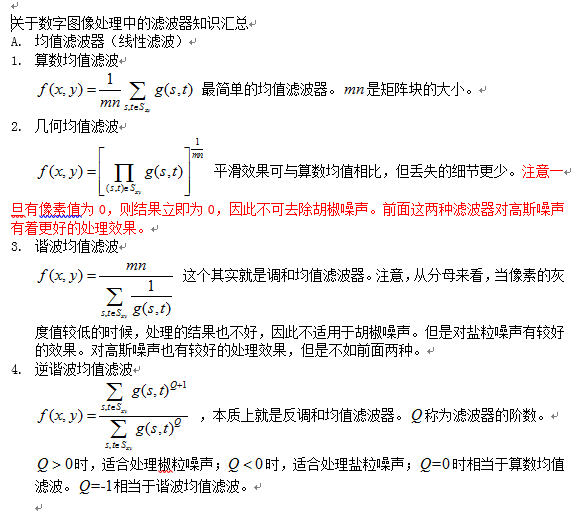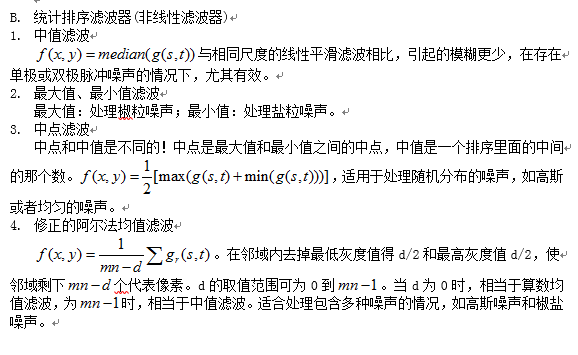展开全文图像处理
• 图像处理滤波器 经过前期的一番准备和学习，我的地盘终于到了我做主的时候。由于我们组的项目和图像处理（运动物体检测与跟踪）有关，和我同组的iamfrankie同学将在FPGA方面对该项目发表一些好的博文，前段时间...
转载地址： http://blog.csdn.net/vast_sea/article/details/8192568

图像处理之滤波器

经过前期的一番准备和学习，我的地盘终于到了我做主的时候。由于我们组的项目和图像处理（运动物体检测与跟踪）有关，和我同组的iamfrankie同学将在FPGA方面对该项目发表一些好的博文，前段时间他的博文也受到各位的好评，其中不乏好的文章，而且有的还被推荐为精品，这里先祝贺他！由于我们组内有分工，他主要负责FPGA技术，我主要负责图像处理算法这块，所以我的文章会以图像处理相关的主题为主。前期我会介绍一些滤波器的知识，我认为这是图像处理中最关键的组成部分之一。无论是在图像变换、图像增强和图像恢复中都是相当重要的。

消除图像中的噪声成分叫作图像的平滑化或滤波操作。信号或图像的能量大部分集中在幅度谱的低频和中频段是很常见的，而在较高频段，感兴趣的信息经常被噪声淹没。因此一个能降低高频成分幅度的滤波器就能够减弱噪声的影响。

滤波的目的有两个:一是抽出对象的特征作为图像识别的特征模式;另一个是为适应图像处理的要求，消除图像数字化时所混入的噪声。

对滤波处理的要求有两条:一是不能损坏图像的轮廓及边缘等重要信息;二是使图像清晰视觉效果好。

图像的滤波方法很多，主要可以分为频率域法和空间域法两大类。频率域法的处理是在图像的某种变换域内，对图像的变换系数值进行运算，然后通过逆变换获得增强图像。这是一种间接的图像滤波方法。空间滤波方法是一类直接的滤波方法，它在处理图像时直接对图像灰度作运算。

频率域滤波是将图像从空间或时间域转换到频率域，再利用变换系数反映某些图像特征的性质进行图像滤波的方法。傅立叶变换是一种常用的变换。在傅立叶变换域，频谱的直流分量正比于图像的平均亮度，噪声对应于频率较高的区域，图像实体位于频率较低的区域。图像在变换具有的这些内在特性可被用于图像滤波。可以构造一个低通滤波器，使低频分量顺利通过而有效地阻于高频分量，即可滤除图像的噪声，再经过反变换来取得平滑的图像。
低通的数学表达式如下式所示:
G(u,v)=F(u,v)H(u,v)

式中F (u, v)一含有噪声的原图像的傅立叶变换;
H (u, v)一为传递函数，也称转移函数（即低通滤波器）;
G (u, v)一为经低通滤波后输出图像的傅立叶变换。
H滤波滤去高频成分，而低频信息基本无损失地通过。滤波后，经傅立叶变换反变换可得平滑图像，即选择适当的传递函数H (u, v)，对频率域低通滤波关系重大。常用的传递函数有梯形函数、指数函数、巴特沃思函数等。
频域常用的几种低通滤波器为理想低通滤波器(Ideal circular Iow-passfilter)、巴特沃思(Butterworth)低通滤波器、指数低通滤波器及梯形低通滤波器。这些低通滤波器，都能在图像内有噪声干扰成分时起到改善的作用。
常用的平面空间域滤波法有两类:一类是拟合图像的方法，包括n阶多项式拟合、离散正交多项式拟合、二次曲面拟合等多种方法;另一类是平滑图像的方法，包括领域平均法、中值滤波法、梯度倒数加权法、选择式掩模法等。

在学习了一些图像处理知识之后，总结成了上一篇博文“图像处理之滤波器”（http://www.openhard.org/myspace/blog/show.php?id=76210），此文主要阐述了为什么要进行滤波和目前滤波方法的大致的分类，也说明了我的博客为什么会偏重于说明图像处理算法。有人可能会说，这个主题放在这个网站的主题好像不符合吧。那我要说说当前数字图像处理的FPGA实现的发展，特别是在进行图像采集和预处理上FPGA有很大的优势。对于计算机视觉、多媒体应用等领域来说，实时的数字图像处理是其中的重点学科之一。传统的数字信号处理算法（如FFT、FIR）大都是ASIC或PDSP构建的，在硬件的实时性上很难满足实时性的要求。FPGA技术将成为数字信号前端的主流，它可以作为并行处理机对图像信号进行预处理从而满足图像处理的实时性。再结合我们团队的《移动物体检测与跟踪系统》欲采用CCD图像传感器采集视频图像。但CCD采集来的图像具有很强的背景噪声，所以就必须要在处理图像前对图像进行滤波，将图像中的噪声滤去。前端预处理系统一般的形式如下图所示。

因此要开发出良好的移动物体检测系统，这一步是必不可少的，这样在后续的处理中可以减少很多不必要的麻烦。既然说了这么多它的必要性和重要性。那么接下来我将总结一下前端时间学习的最主要的高斯滤波和中值滤波两种我认为重要且容易在FPGA上实现的方法。这两种方法分别针对高斯噪声和脉冲噪声两种主要的噪声有很好的效果。

首先是高斯滤波器。 高斯滤波器是平滑线性滤波器的一种，线性滤波器很适合于去除高斯噪声。而非线性滤波则很适合用于去除脉冲噪声，中值滤波就是非线性滤波的一种。平滑滤波器就是用滤波掩模确定的邻域内像素的平均灰度值去替代图像的每个像素点的值，这很容易用硬件实现。而高斯滤波器是带有权重的平均值，即加权平均，中心的权重比邻近像素的权重更大，这样就可以克服边界效应。高斯滤波如果采用3×3掩模的具体公式如下:

g(x,y)={f(x-1,y-1)+f(x-1,y+1)+f(x+1,y-1)+f(x+1,y+1)+[f(x-1,y)+f(x,y-1)+f(x+1,y)+f(x,y+1)]*2+f(x,y)*4}/16;

其中，f(x,y)为原图像中（x,y)像素点的灰度值，g(x,y)为经过高斯滤波和的值。

上述的公式可以结构化为3×3的掩模如下：

×1/16

从结构化掩模中可以看到，处于掩模中心的位置比其他任何像素的权值都大，因此在均值计算中给定的这一像素显得更为重要。而距离掩模中心较远的像素就显得不太重要，这样做是为了减小平滑处理中的模糊。当然可以采取其他的权重达到相同的目的，其中16=1+2+1+2+4+2+1+2+1。但是由于1、2、4、16都是2的整数次幂很便于硬件的实现。

我在网上搜到一篇在FPGA上实现高斯滤波器的具体方法，上传到我的分享中了。

具体的参考数字图像处理的经典书——冈萨雷斯的《数字图像处理（matlab版）》借此机会希望有更多喜欢“图像处理+FPGA”的同学一同探讨这本书上的精华。

其次是中值滤波器。中值滤波器是统计滤波器的一种，属于非线性的的空间滤波器。正如其名，它是将像素（中值计算中包括的原像素值）邻域内灰度的中值代替该像素的值。中值滤波器的使用非常普遍，这是因为对于一定类型的随机噪声，它提供了优秀的去噪能力，比小尺寸的线性平滑滤波器的模糊程度明显要低。中值滤波器对处理脉冲噪声非常有效。中值滤波器在FPGA上实现起来相对高斯滤波的难度更大一点。因为它要对相邻的像素进行排序，需要用到一些比较器。

虽然说中值滤波器对脉冲信号很有效，但是仅采用简单的中值滤波将不可避免的丢失图像的细节，造成视觉效果的模糊。于是后来又些人提出了一些改进的方法，如基于个数判断的脉冲噪声的中值滤波器、自适应门限的中值滤波器、多窗口下的自适应中值滤波器等等。

形态学滤波器
随着数学各分支在理论和应用上的逐步深入，以数学形态学为代表的非线性滤波在保护图像边缘和细节方面取得了显著进展。形态学滤波器是近年来出现的一类重要的非线性滤波器，它由早期的二值形滤波器发展为后来的多值(灰度)形态滤波器，在形状识别、边缘检测、纹理分析、图像恢复和增强等领域了广泛的应用。形态滤波方法充分利用形态学运算所具有的几何特征和良好的代数性质，主要采用态学开、闭运算进行滤波操作。从形态学基本原理可知，形态学的开运算会去掉图像上与结构元素的形态不相吻合的相对亮的分布结构，同时保留那些相吻合的部分;而闭运算则会填充那些图像上与结构元素不相吻合的相对暗的分布结构，同时保留那些相吻合的部分。因此他们都可以用来有效的提取特征和平滑像。值得注意地是，采用形态滤波器时，应根据不同的目的选择具有不同形状、大小和方向特性的结构元素。此外，形态学开、闭运算都具有幂等性，这意味着一次滤波就己将所有特定于结构元素的噪声滤除千净，再次重复不会产生新的结果。这是一个经典方法(如线性卷积滤波、中值滤波)所不具备的性质。由于形态学运算是从图像的几何形态观点来进行图像处理的，因此这种优良的非线性滤波器能在滤波的同时，保持图像结构不被钝化。
展开全文• 描述：均值滤波器图像处理中一种常见的滤波器，它主要应用于平滑噪声。它的原理主要是利用某像素点周边像素的平均值来打到平滑噪声的效果。 常用的均值核如下图所示：   图像滤波器操作实际上就是模板...图像处理 filter image input output
• 滤波器图像处理和计算机视觉中最基础的运算，可以实现很多种不同的图像变换。中值滤波中值滤波（Median filter）是一种典型的非线性滤波技术，基本思想是用像素点邻域灰度值的中值来代替该像素点的灰度值，该方法...
• 如果图像的不同部分使用不同的滤波权重因子，线性滤波器是空间可变的。因此可以使用卷积模板来实现滤波。 线性滤波器对去除高斯噪声有很好的效果。常用的线性滤波器有均值滤波器和高斯平滑滤波器。 (1) 均值滤波器：...图像处理
• 滤波器是根据原有图像的某个像素的周围像素来确定新的像素值，滤波器主要的作用是用来消去噪声的，消除图像中的不合理的像素点。滤波器主要包括线性滤波器和非线性滤波器，其中线性滤波器包括均值滤波，方框滤波和...
• 图像滤波的作用： 在尽可能保留图像细节特征的条件下，对目标图像的噪声进行抑制。 常见的图像滤波器： 线性滤波：均值滤波高斯滤波 非线性滤波：中值滤波双边滤波 *均值滤波 均...
• 数字图像处理 均值滤波器自写函数系统函数参考文件 自写函数 %%自写滤波 I = double(imread('Fig3.35(a).jpg')); [m,n] = size(I); A3 = zeros(m,n); A5 = zeros(m,n); for i = 3:m-3 for j = 3:n-3 A3(i,j) = ( I...均值滤波 数字图像处理
• 高斯滤波器是空间滤波器的一种，学习高斯滤波器，需要空间滤波的知识作为先验知识（各种数字图像处理的书中都有介绍，冈萨雷斯的经典教材说的还是比较清楚的，建议看英文版，个人感觉比翻译过来的更容易理解）。...
• 数字图像处理，评语滤波，理想低通滤波器，高斯滤波器，巴特沃斯滤波器数字图像处理
• 图像增强中，平滑是为了消除图像中噪声的干扰，或者降低对比度，与之相反，有时为了强调图像的边缘和细节，需要对图像进行锐化，提高对比度。 图的边缘是指在局部不连续的特征。 简要介绍一下原理： 拉普拉斯...
• 几乎所有真正有价值的图像降噪方法都在试图让程序可以...目前这样的方法已有很多，例如比较有名的双边滤波、各向异性扩散滤波和基于TV的去噪方法等等，而且它们在医学图像处理或者美颜嫩肤算法等领域中都有重要应用图像处理 维纳滤波 MATLAB
• 滤波器是根据原有图像的某个像素的周围像素来确定新的像素值，滤波器主要的作用是用来消去噪声的，消除图像中的不合理的像素点。滤波器主要包括线性滤波器和非线性滤波器，其中线性滤波器包括均值滤波，方框滤波和...opencv
• 为什么说在数字图像处理中使用陷波滤波器来对一幅图像的DFT进行修改时不需要考虑填充呢？虽说陷波滤波器有其相应的公式，不过他也是可以通过高通滤波器的乘积而得到的，不过在进行高通滤波时就需要考虑填充了，所以...人工智能
• 高斯滤波器是一种线性滤波器，能够有效的抑制噪声，平滑图像。其作用原理和均值滤波器类似，都是取滤波器窗口内的像素的均值作为输出。其窗口模板的系数和均值滤波器不同，均值滤波器的模板系数都是相同的为1；而...
...